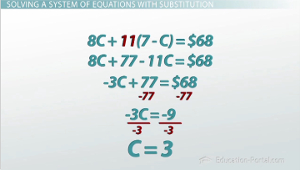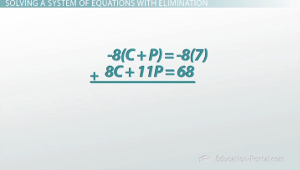# How Do I Use a System of Equations?

An error occurred trying to load this video.

Try refreshing the page, or contact customer support.

Coming up next: What is a Matrix?

### You're on a roll. Keep up the good work!

Replay
Your next lesson will play in 10 seconds
• 0:05 Building a System of Equations
• 4:12 Solving with Substitution
• 6:17 Solving with Elimination
• 8:16 Lesson Summary

Want to watch this again later?

Timeline
Autoplay
Autoplay
Speed

#### Recommended Lessons and Courses for You

Lesson Transcript
Instructor: Jeff Calareso

Jeff teaches high school English, math and other subjects. He has a master's degree in writing and literature.

There are a few classic algebra word problems, such as the one about two trains traveling at different speeds. In this lesson, you'll learn how to take a word problem and convert it into the system of equations that will allow you to find the answer using either substitution or elimination.

## Building a System of Equations

Probably the best known algebra word problem (which often seems to have been a traumatic experience for people) has to do with two trains leaving the station going opposite directions. It usually asks something along the lines of how fast they were going or how far apart they were. But I like to make math as un-traumatic as possible, so we're going to stay away from any train problems and instead, we're going to look at a difference classic word problem.

Let's say you're hosting a Super Bowl party and have been given \$68 by all your friends that are coming to spend on pizza. You're going to get either cheese pizza or pepperoni pizza. Cheese pizzas cost \$8 each, and the pepperoni pizzas cost \$11 each. Since you might as well spend all the money (it's your friends', not yours) and you've also decided that 7 pizzas is the perfect amount of food, the question is how many of the seven pizzas should be cheese, and how many of them should be pepperoni?

We could try to guess and check our way through this problem, which might work for this one, but that's probably not going to work for most problems like this. So we'd rather use this example as a chance to learn how to do it with math. But in order to do it with math, we have to first change the words into mathematical equations. This can oftentimes be the hardest part.

In order to set up those equations, we need to figure out what the variables we're looking for are. In order to that, we're going to have to go back to the problem and figure out what it's really asking us for. This problem is asking us for how many cheese pizzas and how many pepperoni pizzas should we buy. Which means our variables should be the number of cheese pizzas (C) and the number of pepperoni pizzas (P). When we solve for those two things, we'll know how many we should buy.

So now that we have our variables, we have to come up with equations that represent the situation. This problem is going to need two equations to solve it because there are two variables, which means that this is a system of equations, because there's going to be more than one.

The easier equation to come up with uses the fact that we know we have to buy a total of 7 pizzas. We've decided that 7 pizzas is the perfect amount. Since C is the number of cheese and P is the number of pepperoni, and we know that the total has to be 7, my first equation is the number of cheese (C) plus the number of pepperoni (P) must equal 7: C + P = 7.

The harder equation to come up with has to do with the price and how much money we have and how much money different pizzas cost. What I want to do is set up an equation that equals 68. It should say that we are going to spend \$68. This means I have to figure out how much money I'm spending on my cheese pizzas and how much money I'm spending on my pepperoni pizzas.Well, if we just think about this logically, if I bought one cheese pizza, it would cost me \$8. And if I bought two, it would cost me \$16. If I bought three, it would cost me \$24, and so on. This means that the total money I spend on my cheese pizzas is simply 8 times the number of cheese pizzas I buy (8C). A very similar expression could be derived for the amount of money you spend on pepperoni pizzas by doing 11 times the number of pepperoni pizzas you buy (11P).

So 8C represents the money you spend on cheese pizzas and 11P represents the money you spend on pepperoni pizzas. If you add those two amounts together, that's the total amount we spend. And that has to be \$68. So our second equation, the little more complicated one, is 8C + 11P = \$68.

## Solving a System of Equations with Substitution

Now that we have the system set up, we can actually begin to solve it. We're going to go over 2 ways of solving a system of equations, the first of which is called substitution. It is called this because we're going to take one expression from one equation and substitute it in for one of the variables in our second equation.

For example, we can take the equation that says C + P = 7, telling us the total number of pizzas we're getting, and we can solve this equation for P by undoing C from both sides with subtraction. We find an equivalent equation that says that P = 7 - C.

Now that I know what P equals, and since I want these two equations to be the same, I can substitute into the second equation what I now know what P is. So instead of writing 8C + 11P = \$68, I can write 8C + 11(7 - C) = \$68. Now I only have one variable in one equation, which is solvable for that variable.To unlock this lesson you must be a Study.com Member.

### Register to view this lesson

Are you a student or a teacher?

### Unlock Your Education

#### See for yourself why 30 million people use Study.com

##### Become a Study.com member and start learning now.
Back
What teachers are saying about Study.com

### Earning College Credit

Did you know… We have over 160 college courses that prepare you to earn credit by exam that is accepted by over 1,500 colleges and universities. You can test out of the first two years of college and save thousands off your degree. Anyone can earn credit-by-exam regardless of age or education level.